Share

Books Shortlist
Your shortlist is empty

# RD Sharma solutions for Class 9 Mathematics chapter 16 - Circles

## Chapter 16 : Circles

#### Page 0

Fill in the blank:

All points lying inside/outside a circle are called .................. points/ .....................points.

Fill in the blank

Circles having the same centre and different radii are called ...........................circles.

Fill in the blank:

A point whose distance from the centre of a circle is greater than its radius lies in ..................... of the circle.

Fill in the blank

A continuous piece of a circle is ............... of the circle

Fill in the blank:

The longest chord of a circle is a ................of the circle.

Fill in the blank:

An arc is a ................ when its ends are the ends of a diameter.

Fill in the blank:

Segment of a circle is the region between an arc and .................. of the circle.

Fill in the blank:

A circle divides the plane, on which it lies, in ............ parts.

true or false

A circle is a plane figure.

true or false

Line segment joining the centre to any point on the circle is a radius of the circle,

true or false

If a circle is divided into three equal arcs each is a major arc.

true or false

A circle has only finite number of equal chords.

true or false

A chord of a circle, which is twice as long is its radius is a diameter of the circle.

true or false

Sector is the region between the chord and its corresponding arc.

true or false

The degree measure of an arc is the complement of the central angle containing the arc.

ture or false v

The degree measure of a semi-circle is 180°.

#### Page 0

The radius of a circle is 8 cm and the length of one of its chords is 12 cm. Find the distance of the chord from the centre.

Find the length of a chord which is at a distance of 5 cm from the centre of a circle ofradius 10 cm.

Find the length of a chord which is at a distance of 4 cm from the centre of the circle of radius 6 cm.

Two chords AB, CD of lengths 5 cm, 11 cm respectively of a circle are parallel. If the distance between AB and CD is 3 cm, find the radius of the circle.

Give a method to find the centre of a given circle.

Prove that the line joining the mid-point of a chord to the centre of the circle passes through the mid-point of the corresponding minor arc.

Prove that a diameter of a circle which bisects a chord of the circle also bisects the angle subtended by the chord at the centre of the circle.

Given an arc of a circle, show how to complete the circle.

Prove that two different circles cannot intersect each other at more than two points.

A line segment AB is of length 5 cm. Draw a circle of radius 4 cm passing through A and B. Can you draw a circle of radius 2 cm passing through A and B? Give reason in support of your answer.

An equilateral triangle of side 9cm is inscribed in a circle. Find the radius of the circle.

Given an arc of a circle, complete the circle.

Draw different pairs of circles. How many points does each pair have in common? What is the maximum number of common points?

Suppose You Are Given a Circle. Give a Construction to Find Its Centre.

Two chords AB and CD of lengths 5 cm and 11 cm respectively of a circle are parallel to each other and are opposite side of its center. If the distance between AB and CD is 6 cm. Find the radius of the circle.

The lengths of two parallel chords of a circle are 6 cm and 8 cm. if the smaller chord is at a distance of 4 cm from the centre, what is the distance of the other chord from the centre?

#### Page 0

Three girls Ishita, Isha and Nisha are playing a game by standing on a circle of radius 20 m drawn in a park. Ishita throws a ball o Isha, Isha to Nisha and Nisha to Ishita. If the distance between Ishita and Isha and between Isha and Nisha is 24 m each, what is the distance between Ishita and Nisha.

A circular park of radius 40 m is situated in a colony. Three boys Ankur, Amit and Anand are sitting at equal distance on its boundary each having a toy telephone in his hands to talk
to each other.

#### Page 0

In the below fig. O is the centre of the circle. If ∠APB = 50°, find ∠AOB and ∠OAB.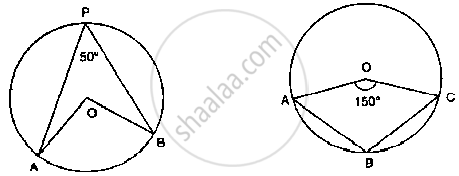In the fig below, it is given that O is the centre of the circle and ∠AOC = 150°. Find
∠ABC.In the below fig. O is the centre of the circle. Find ∠BAC.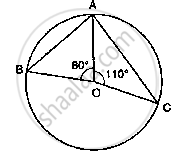If O is the centre of the circle, find the value of x in the following figure: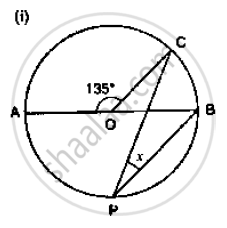If O is the centre of the circle, find the value of x in the following figure: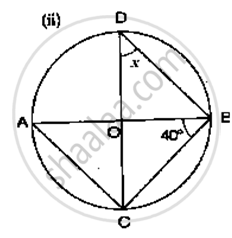If O is the centre of the circle, find the value of x in the following figure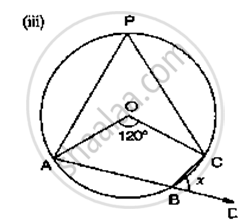If O is the centre of the circle, find the value of x in the following figure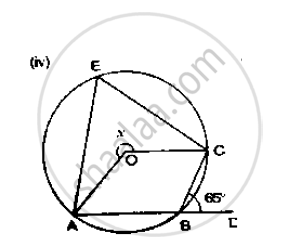If O is the centre of the circle, find the value of x in the following figure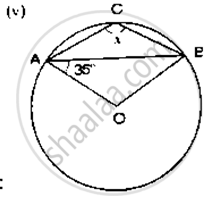If O is the centre of the circle, find the value of x in the following figure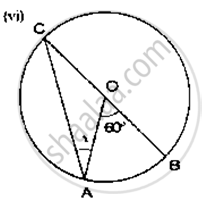If O is the centre of the circle, find the value of x in the following figure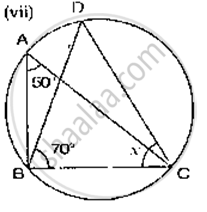If O is the centre of the circle, find the value of x in the following figure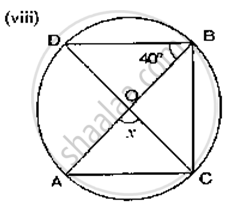If O is the centre of the circle, find the value of x in the following figure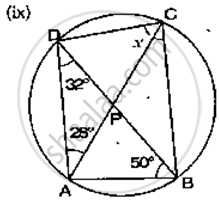If O is the centre of the circle, find the value of x in the following figure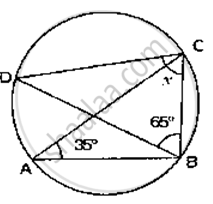## RD Sharma solutions for Class 9 Mathematics chapter 16 - Circles

RD Sharma solutions for Class 9 Maths chapter 16 (Circles) include all questions with solution and detail explanation. This will clear students doubts about any question and improve application skills while preparing for board exams. The detailed, step-by-step solutions will help you understand the concepts better and clear your confusions, if any. Shaalaa.com has the CBSE Mathematics for Class 9 by R D Sharma (2018-19 Session) solutions in a manner that help students grasp basic concepts better and faster.

Further, we at shaalaa.com are providing such solutions so that students can prepare for written exams. RD Sharma textbook solutions can be a core help for self-study and acts as a perfect self-help guidance for students.

Concepts covered in Class 9 Mathematics chapter 16 Circles are Cyclic Quadrilaterals, Angle Subtended by an Arc of a Circle, Equal Chords and Their Distances from the Centre, Circle Through Three Points, Perpendicular from the Centre to a Chord, Angle Subtended by a Chord at a Point, Circles and Its Related Terms.

Using RD Sharma Class 9 solutions Circles exercise by students are an easy way to prepare for the exams, as they involve solutions arranged chapter-wise also page wise. The questions involved in RD Sharma Solutions are important questions that can be asked in the final exam. Maximum students of CBSE Class 9 prefer RD Sharma Textbook Solutions to score more in exam.

Get the free view of chapter 16 Circles Class 9 extra questions for Maths and can use shaalaa.com to keep it handy for your exam preparation

S